# matplotlib.gridspec.GridSpec¶

class matplotlib.gridspec.GridSpec(nrows, ncols, figure=None, left=None, bottom=None, right=None, top=None, wspace=None, hspace=None, width_ratios=None, height_ratios=None)[source]

Specifies the geometry of the grid that a subplot can be placed in.

The location of grid is determined by similar way as the SubplotParams.

The number of rows and number of columns of the grid need to be set. Optionally, the subplot layout parameters (e.g., left, right, etc.) can be tuned.

Parameters: nrows : int Number of rows in grid. ncols : int Number or columns in grid. figure : Figure, optional left, right, top, bottom : float, optional Extent of the subplots as a fraction of figure width or height. Left cannot be larger than right, and bottom cannot be larger than top. wspace : float, optional The amount of width reserved for space between subplots, expressed as a fraction of the average axis width. hspace : float, optional The amount of height reserved for space between subplots, expressed as a fraction of the average axis height. width_ratios : length ncols iterable, optional Width ratios of the columns. height_ratios : length nrows iterable, optional Height ratios of the rows.

Notes

See SubplotParams for descriptions of the layout parameters.

get_subplot_params(self, figure=None)[source]

Return a dictionary of subplot layout parameters. The default parameters are from rcParams unless a figure attribute is set.

locally_modified_subplot_params(self)[source]
tight_layout(self, figure, renderer=None, pad=1.08, h_pad=None, w_pad=None, rect=None)[source]

Parameters: pad : float Padding between the figure edge and the edges of subplots, as a fraction of the font-size. h_pad, w_pad : float, optional Padding (height/width) between edges of adjacent subplots. Defaults to pad. rect : tuple of 4 floats, optional (left, bottom, right, top) rectangle in normalized figure coordinates that the whole subplots area (including labels) will fit into. Default is (0, 0, 1, 1).
update(self, **kwargs)[source]

Update the current values.

Values set to None use the rcParams value.

## Examples using matplotlib.gridspec.GridSpec¶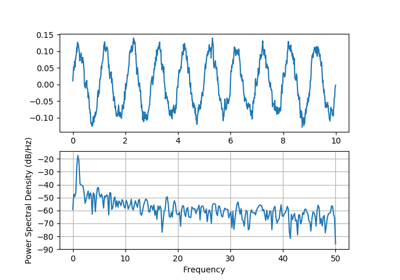Psd Demo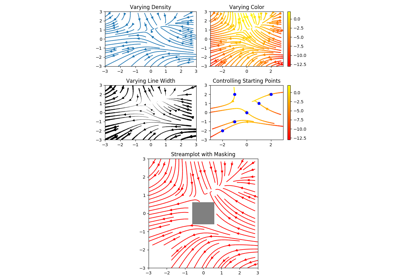Streamplot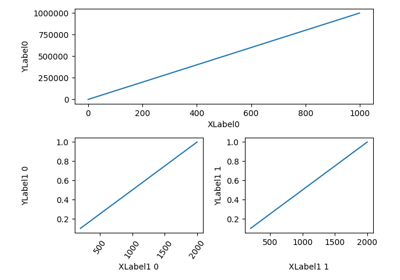Aligning Labels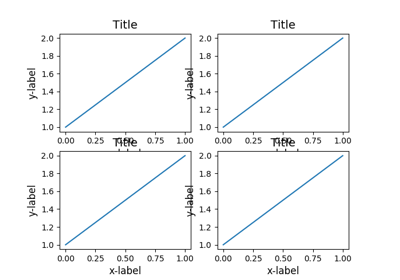Resizing axes with constrained layout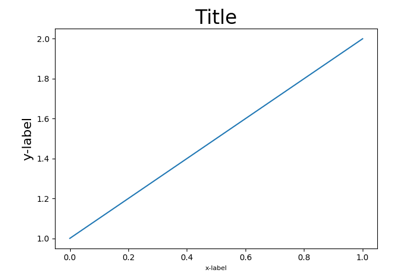Resizing axes with tight layout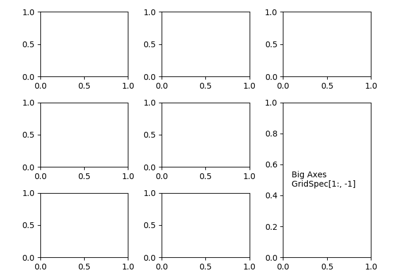Combining two subplots using subplots and GridSpec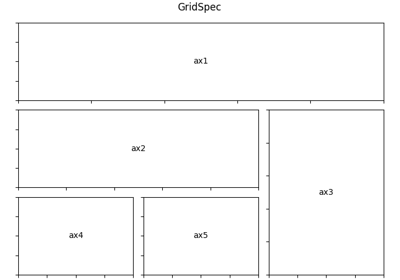Using Gridspec to make multi-column/row subplot layouts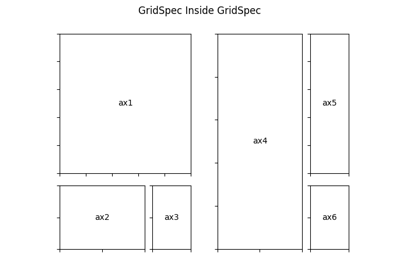Nested Gridspecs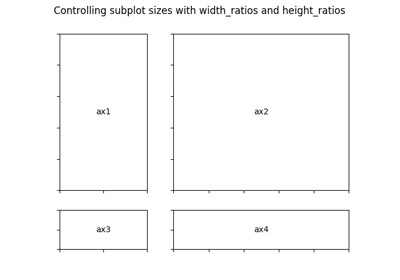GridSpec demo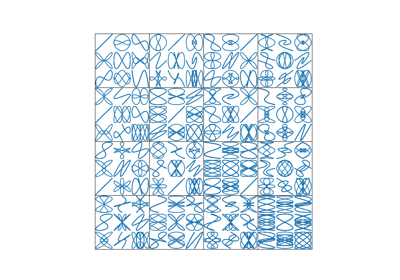Nested GridSpecs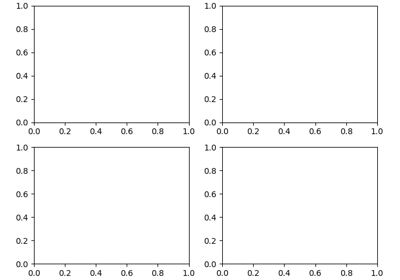Customizing Figure Layouts Using GridSpec and Other Functions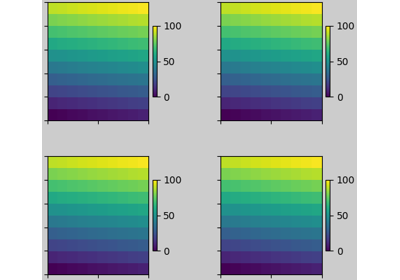Constrained Layout Guide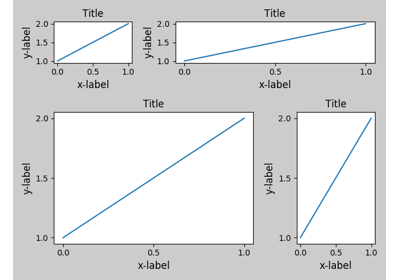Tight Layout guide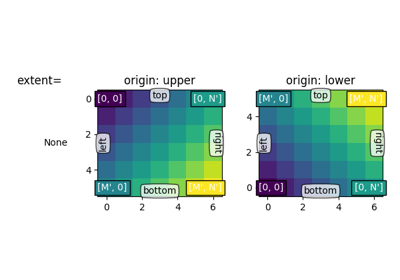origin and extent in imshow